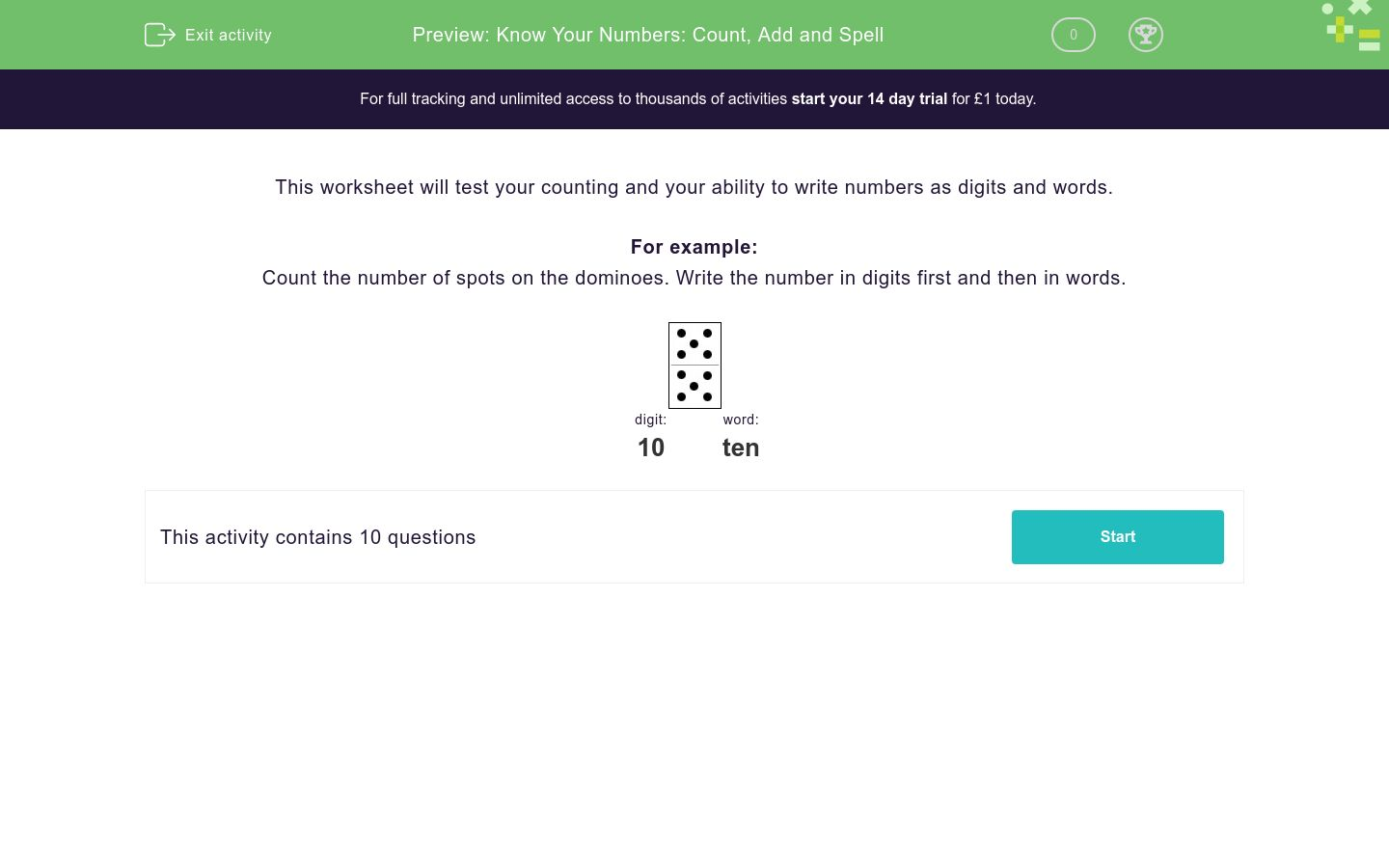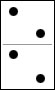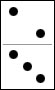### Affordable pricing from £10/month

In this worksheet, students do a simple addition, then they write their answer in both numbers and words.Key stage:  KS 1

Curriculum topic:   Number: Number and Place Value

Curriculum subtopic:   Read and Write Numerals to 100 (including multiples of 2, 5 and 10)

Difficulty level:### QUESTION 1 of 10

This worksheet will test your counting and your ability to write numbers as digits and words.

For example:

Count the number of spots on the dominoes. Write the number in digits first and then in words.digit: word: 10 ten

Count the number of spots on the dominoes and write the number in digits first and then in words.digit word number

Count the number of spots on the dominoes and write the number in digits first and then in words.digit word number

Count the number of spots on the dominoes and write the number in digits first and then in words.digit word number

Count the number of spots on the dominoes and write the number in digits first and then in words.digit word number

Count the number of spots on the dominoes and write the number in digits first and then in words.digit word number

Count the number of spots on the dominoes and write the number in digits first and then in words.digit word number

Count the number of spots on the dominoes and write the number in digits first and then in words.digit word number

Count the number of spots on the dominoes and write the number in digits first and then in words.digit word number

Count the number of spots on the dominoes and write the number in digits first and then in words.digit word number

Count the number of spots on the dominoes and write the number in digits first and then in words.digit word number
• Question 1

Count the number of spots on the dominoes and write the number in digits first and then in words.digit word number
EDDIE SAYS
Four = 4
• Question 2

Count the number of spots on the dominoes and write the number in digits first and then in words.digit word number
EDDIE SAYS
Seven = 7
• Question 3

Count the number of spots on the dominoes and write the number in digits first and then in words.digit word number
EDDIE SAYS
Nine = 9
• Question 4

Count the number of spots on the dominoes and write the number in digits first and then in words.digit word number
EDDIE SAYS
One = 1
• Question 5

Count the number of spots on the dominoes and write the number in digits first and then in words.digit word number
EDDIE SAYS
Three = 3
• Question 6

Count the number of spots on the dominoes and write the number in digits first and then in words.digit word number
EDDIE SAYS
Eight = 8
• Question 7

Count the number of spots on the dominoes and write the number in digits first and then in words.digit word number
EDDIE SAYS
Ten = 10
• Question 8

Count the number of spots on the dominoes and write the number in digits first and then in words.digit word number
EDDIE SAYS
Five = 5
• Question 9

Count the number of spots on the dominoes and write the number in digits first and then in words.digit word number
EDDIE SAYS
Six = 6
• Question 10

Count the number of spots on the dominoes and write the number in digits first and then in words.digit word number
EDDIE SAYS
Eleven = 11
---- OR ----

Sign up for a £1 trial so you can track and measure your child's progress on this activity.

### What is EdPlace?

We're your National Curriculum aligned online education content provider helping each child succeed in English, maths and science from year 1 to GCSE. With an EdPlace account you’ll be able to track and measure progress, helping each child achieve their best. We build confidence and attainment by personalising each child’s learning at a level that suits them.

Get started